# Mean State

Period Mean (original grids) [Watt m-2]
Model Period Mean (intersection) [Watt m-2]
Model Period Mean (complement) [Watt m-2]
Benchmark Period Mean (intersection) [Watt m-2]
Benchmark Period Mean (complement) [Watt m-2]
Bias [Watt m-2]
RMSE [Watt m-2]
Phase Shift [months]
Bias Score 
RMSE Score 
Seasonal Cycle Score 
Spatial Distribution Score 
Interannual Variability Score 
Overall Score 
Benchmark [-] 453.
CLM4 [-] 456. 456. 0.00 453. 460. 3.92 12.5 1.52 0.55 0.37 0.78 1.0 0.76 0.64
CLM4.5 [-] 460. 461. 0.00 453. 460. 8.11 15.4 1.46 0.41 0.37 0.79 1.0 0.74 0.61
CLM5 [-] 462. 462. 0.00 453. 460. 9.34 15.7 1.72 0.34 0.37 0.75 0.99 0.71 0.59
Period Mean (original grids) [Watt m-2]
Model Period Mean (intersection) [Watt m-2]
Model Period Mean (complement) [Watt m-2]
Benchmark Period Mean (intersection) [Watt m-2]
Benchmark Period Mean (complement) [Watt m-2]
Bias [Watt m-2]
RMSE [Watt m-2]
Phase Shift [months]
Bias Score 
RMSE Score 
Seasonal Cycle Score 
Spatial Distribution Score 
Interannual Variability Score 
Overall Score 
Benchmark [-] 471.
CLM4 [-] 474. 475. 0.00 471. 456. 3.22 17.5 0.681 0.69 0.50 0.95 1.0 0.76 0.73
CLM4.5 [-] 480. 480. 0.00 471. 456. 8.58 20.4 0.621 0.57 0.51 0.95 0.94 0.74 0.70
CLM5 [-] 481. 482. 0.00 471. 456. 10.1 20.9 0.713 0.56 0.50 0.95 0.95 0.71 0.69
Period Mean (original grids) [Watt m-2]
Model Period Mean (intersection) [Watt m-2]
Model Period Mean (complement) [Watt m-2]
Benchmark Period Mean (intersection) [Watt m-2]
Benchmark Period Mean (complement) [Watt m-2]
Bias [Watt m-2]
RMSE [Watt m-2]
Phase Shift [months]
Bias Score 
RMSE Score 
Seasonal Cycle Score 
Spatial Distribution Score 
Interannual Variability Score 
Overall Score 
Benchmark [-] 443.
CLM4 [-] 446. 446. 0.00 443. 444. 2.74 15.3 0.853 0.66 0.51 0.90 0.99 0.65 0.70
CLM4.5 [-] 452. 452. 0.00 443. 444. 8.38 18.2 0.844 0.53 0.51 0.90 0.98 0.65 0.68
CLM5 [-] 451. 451. 0.00 443. 444. 7.63 17.9 1.14 0.57 0.49 0.85 0.99 0.61 0.67
Period Mean (original grids) [Watt m-2]
Model Period Mean (intersection) [Watt m-2]
Model Period Mean (complement) [Watt m-2]
Benchmark Period Mean (intersection) [Watt m-2]
Benchmark Period Mean (complement) [Watt m-2]
Bias [Watt m-2]
RMSE [Watt m-2]
Phase Shift [months]
Bias Score 
RMSE Score 
Seasonal Cycle Score 
Spatial Distribution Score 
Interannual Variability Score 
Overall Score 
Benchmark [-] 276.
CLM4 [-] 274. 274. 0.00 275. 292. 1.58 15.1 0.0560 0.91 0.80 1.0 1.0 0.82 0.89
CLM4.5 [-] 274. 274. 0.00 275. 292. 1.52 15.1 0.0575 0.91 0.80 1.0 0.99 0.80 0.89
CLM5 [-] 275. 275. 0.00 275. 292. 3.64 16.0 0.0557 0.90 0.80 1.0 0.99 0.79 0.88
Period Mean (original grids) [Watt m-2]
Model Period Mean (intersection) [Watt m-2]
Model Period Mean (complement) [Watt m-2]
Benchmark Period Mean (intersection) [Watt m-2]
Benchmark Period Mean (complement) [Watt m-2]
Bias [Watt m-2]
RMSE [Watt m-2]
Phase Shift [months]
Bias Score 
RMSE Score 
Seasonal Cycle Score 
Spatial Distribution Score 
Interannual Variability Score 
Overall Score 
Benchmark [-] 456.
CLM4 [-] 451. 451. 0.00 453. 465. -0.292 8.68 1.84 0.38 0.39 0.72 0.92 0.71 0.58
CLM4.5 [-] 454. 454. 0.00 453. 465. 1.47 9.23 1.82 0.35 0.39 0.72 0.92 0.71 0.58
CLM5 [-] 458. 458. 0.00 453. 465. 5.84 10.1 1.76 0.31 0.41 0.73 0.93 0.72 0.59
Period Mean (original grids) [Watt m-2]
Model Period Mean (intersection) [Watt m-2]
Model Period Mean (complement) [Watt m-2]
Benchmark Period Mean (intersection) [Watt m-2]
Benchmark Period Mean (complement) [Watt m-2]
Bias [Watt m-2]
RMSE [Watt m-2]
Phase Shift [months]
Bias Score 
RMSE Score 
Seasonal Cycle Score 
Spatial Distribution Score 
Interannual Variability Score 
Overall Score 
Benchmark [-] 306.
CLM4 [-] 312. 312. 0.00 306. 329. 6.72 13.0 0.0202 0.90 0.86 1.0 1.0 0.89 0.92
CLM4.5 [-] 313. 312. 0.00 306. 329. 7.02 13.1 0.0199 0.90 0.86 1.0 1.0 0.90 0.92
CLM5 [-] 316. 315. 0.00 306. 329. 10.1 14.8 0.0215 0.86 0.86 1.0 1.0 0.89 0.91
Period Mean (original grids) [Watt m-2]
Model Period Mean (intersection) [Watt m-2]
Model Period Mean (complement) [Watt m-2]
Benchmark Period Mean (intersection) [Watt m-2]
Benchmark Period Mean (complement) [Watt m-2]
Bias [Watt m-2]
RMSE [Watt m-2]
Phase Shift [months]
Bias Score 
RMSE Score 
Seasonal Cycle Score 
Spatial Distribution Score 
Interannual Variability Score 
Overall Score 
Benchmark [-] 362.
CLM4 [-] 360. 360. 0.00 361. 395. 1.19 14.3 0.102 0.88 0.82 0.99 0.98 0.75 0.87
CLM4.5 [-] 361. 360. 0.00 361. 395. 1.65 14.2 0.0991 0.88 0.82 0.99 0.98 0.74 0.87
CLM5 [-] 364. 363. 0.00 361. 395. 4.75 15.4 0.151 0.86 0.82 0.99 0.99 0.73 0.87
Period Mean (original grids) [Watt m-2]
Model Period Mean (intersection) [Watt m-2]
Model Period Mean (complement) [Watt m-2]
Benchmark Period Mean (intersection) [Watt m-2]
Benchmark Period Mean (complement) [Watt m-2]
Bias [Watt m-2]
RMSE [Watt m-2]
Phase Shift [months]
Bias Score 
RMSE Score 
Seasonal Cycle Score 
Spatial Distribution Score 
Interannual Variability Score 
Overall Score 
Benchmark [-] 450.
CLM4 [-] 445. 446. 0.00 450. 463. -1.23 16.8 0.656 0.69 0.55 0.93 1.0 0.67 0.73
CLM4.5 [-] 450. 451. 0.00 450. 463. 2.82 17.5 0.654 0.66 0.56 0.93 1.0 0.66 0.73
CLM5 [-] 452. 452. 0.00 450. 463. 4.66 17.7 0.767 0.65 0.56 0.92 1.0 0.63 0.72
Period Mean (original grids) [Watt m-2]
Model Period Mean (intersection) [Watt m-2]
Model Period Mean (complement) [Watt m-2]
Benchmark Period Mean (intersection) [Watt m-2]
Benchmark Period Mean (complement) [Watt m-2]
Bias [Watt m-2]
RMSE [Watt m-2]
Phase Shift [months]
Bias Score 
RMSE Score 
Seasonal Cycle Score 
Spatial Distribution Score 
Interannual Variability Score 
Overall Score 
Benchmark [-] 275.
CLM4 [-] 280. 280. 0.00 275. 280. 6.13 14.0 0.0290 0.89 0.82 1.0 1.0 0.87 0.90
CLM4.5 [-] 279. 280. 0.00 275. 280. 5.62 13.6 0.0329 0.90 0.83 1.0 1.0 0.86 0.90
CLM5 [-] 282. 282. 0.00 275. 280. 7.91 15.3 0.0355 0.87 0.82 1.0 1.0 0.85 0.89
Period Mean (original grids) [Watt m-2]
Model Period Mean (intersection) [Watt m-2]
Model Period Mean (complement) [Watt m-2]
Benchmark Period Mean (intersection) [Watt m-2]
Benchmark Period Mean (complement) [Watt m-2]
Bias [Watt m-2]
RMSE [Watt m-2]
Phase Shift [months]
Bias Score 
RMSE Score 
Seasonal Cycle Score 
Spatial Distribution Score 
Interannual Variability Score 
Overall Score 
Benchmark [-] 448.
CLM4 [-] 447. 447. 0.00 450. 423. -5.23 14.5 0.334 0.77 0.72 0.97 1.0 0.73 0.82
CLM4.5 [-] 448. 448. 0.00 450. 423. -4.68 14.6 0.329 0.76 0.72 0.97 1.0 0.72 0.82
CLM5 [-] 449. 450. 0.00 450. 423. -3.08 14.6 0.337 0.78 0.71 0.97 0.99 0.70 0.81
Period Mean (original grids) [Watt m-2]
Model Period Mean (intersection) [Watt m-2]
Model Period Mean (complement) [Watt m-2]
Benchmark Period Mean (intersection) [Watt m-2]
Benchmark Period Mean (complement) [Watt m-2]
Bias [Watt m-2]
RMSE [Watt m-2]
Phase Shift [months]
Bias Score 
RMSE Score 
Seasonal Cycle Score 
Spatial Distribution Score 
Interannual Variability Score 
Overall Score 
Benchmark [-] 364.
CLM4 [-] 360. 360. 0.00 362. 385. 1.09 10.8 0.244 0.87 0.80 0.98 0.99 0.84 0.88
CLM4.5 [-] 359. 359. 0.00 362. 385. 0.572 10.1 0.218 0.88 0.81 0.99 0.99 0.84 0.89
CLM5 [-] 363. 363. 0.00 362. 385. 3.66 10.8 0.476 0.86 0.81 0.97 0.99 0.83 0.88
Period Mean (original grids) [Watt m-2]
Model Period Mean (intersection) [Watt m-2]
Model Period Mean (complement) [Watt m-2]
Benchmark Period Mean (intersection) [Watt m-2]
Benchmark Period Mean (complement) [Watt m-2]
Bias [Watt m-2]
RMSE [Watt m-2]
Phase Shift [months]
Bias Score 
RMSE Score 
Seasonal Cycle Score 
Spatial Distribution Score 
Interannual Variability Score 
Overall Score 
Benchmark [-] 397.
CLM4 [-] 375. 375. 0.00 376. 406. 0.774 14.9 0.471 0.75 0.68 0.95 1.0 0.76 0.80
CLM4.5 [-] 377. 377. 0.00 376. 406. 2.18 15.4 0.462 0.72 0.69 0.95 1.0 0.75 0.80
CLM5 [-] 380. 380. 0.00 376. 406. 4.60 16.3 0.517 0.70 0.68 0.94 1.0 0.74 0.79
Period Mean (original grids) [Watt m-2]
Model Period Mean (intersection) [Watt m-2]
Model Period Mean (complement) [Watt m-2]
Benchmark Period Mean (intersection) [Watt m-2]
Benchmark Period Mean (complement) [Watt m-2]
Bias [Watt m-2]
RMSE [Watt m-2]
Phase Shift [months]
Bias Score 
RMSE Score 
Seasonal Cycle Score 
Spatial Distribution Score 
Interannual Variability Score 
Overall Score 
Benchmark [-] 464.
CLM4 [-] 448. 448. 0.00 465. 441. -17.1 24.8 0.424 0.69 0.74 0.97 0.98 0.66 0.79
CLM4.5 [-] 448. 448. 0.00 465. 441. -16.7 24.5 0.415 0.70 0.74 0.97 0.98 0.66 0.79
CLM5 [-] 450. 449. 0.00 465. 441. -15.4 24.1 0.443 0.71 0.73 0.97 0.97 0.64 0.79
Period Mean (original grids) [Watt m-2]
Model Period Mean (intersection) [Watt m-2]
Model Period Mean (complement) [Watt m-2]
Benchmark Period Mean (intersection) [Watt m-2]
Benchmark Period Mean (complement) [Watt m-2]
Bias [Watt m-2]
RMSE [Watt m-2]
Phase Shift [months]
Bias Score 
RMSE Score 
Seasonal Cycle Score 
Spatial Distribution Score 
Interannual Variability Score 
Overall Score 
Benchmark [-] 278.
CLM4 [-] 283. 283. 0.00 277. 289. 5.82 12.9 0.0629 0.89 0.83 1.0 0.99 0.83 0.90
CLM4.5 [-] 283. 282. 0.00 277. 289. 5.52 12.8 0.0535 0.89 0.83 1.0 1.0 0.82 0.90
CLM5 [-] 285. 285. 0.00 277. 289. 8.39 14.5 0.0541 0.86 0.83 1.0 0.99 0.82 0.89
Period Mean (original grids) [Watt m-2]
Model Period Mean (intersection) [Watt m-2]
Model Period Mean (complement) [Watt m-2]
Benchmark Period Mean (intersection) [Watt m-2]
Benchmark Period Mean (complement) [Watt m-2]
Bias [Watt m-2]
RMSE [Watt m-2]
Phase Shift [months]
Bias Score 
RMSE Score 
Seasonal Cycle Score 
Spatial Distribution Score 
Interannual Variability Score 
Overall Score 
Benchmark [-] 444.
CLM4 [-] 445. 445. 0.00 442. 455. 7.04 14.7 0.596 0.55 0.58 0.94 0.94 0.74 0.72
CLM4.5 [-] 445. 445. 0.00 442. 455. 6.38 14.1 0.610 0.58 0.58 0.93 0.98 0.73 0.73
CLM5 [-] 446. 446. 0.00 442. 455. 7.88 14.4 0.674 0.55 0.58 0.93 0.99 0.72 0.73
Period Mean (original grids) [Watt m-2]
Model Period Mean (intersection) [Watt m-2]
Model Period Mean (complement) [Watt m-2]
Benchmark Period Mean (intersection) [Watt m-2]
Benchmark Period Mean (complement) [Watt m-2]
Bias [Watt m-2]
RMSE [Watt m-2]
Phase Shift [months]
Bias Score 
RMSE Score 
Seasonal Cycle Score 
Spatial Distribution Score 
Interannual Variability Score 
Overall Score 
Benchmark [-] 386.
CLM4 [-] 384. 384. 0.00 384. 429. 2.59 8.87 0.141 0.91 0.85 0.99 1.0 0.88 0.91
CLM4.5 [-] 386. 386. 0.00 384. 429. 4.32 9.66 0.149 0.88 0.85 0.99 1.0 0.88 0.91
CLM5 [-] 390. 390. 0.00 384. 429. 8.58 11.9 0.176 0.83 0.86 0.99 1.0 0.86 0.90
Period Mean (original grids) [Watt m-2]
Model Period Mean (intersection) [Watt m-2]
Model Period Mean (complement) [Watt m-2]
Benchmark Period Mean (intersection) [Watt m-2]
Benchmark Period Mean (complement) [Watt m-2]
Bias [Watt m-2]
RMSE [Watt m-2]
Phase Shift [months]
Bias Score 
RMSE Score 
Seasonal Cycle Score 
Spatial Distribution Score 
Interannual Variability Score 
Overall Score 
Benchmark [-] 319.
CLM4 [-] 324. 324. 0.00 318. 343. 6.95 12.4 0.0825 0.87 0.84 0.99 0.99 0.85 0.90
CLM4.5 [-] 324. 324. 0.00 318. 343. 6.93 12.6 0.0830 0.87 0.84 0.99 0.99 0.84 0.90
CLM5 [-] 327. 327. 0.00 318. 343. 10.1 14.6 0.138 0.83 0.84 0.99 0.99 0.84 0.89
Period Mean (original grids) [Watt m-2]
Model Period Mean (intersection) [Watt m-2]
Model Period Mean (complement) [Watt m-2]
Benchmark Period Mean (intersection) [Watt m-2]
Benchmark Period Mean (complement) [Watt m-2]
Bias [Watt m-2]
RMSE [Watt m-2]
Phase Shift [months]
Bias Score 
RMSE Score 
Seasonal Cycle Score 
Spatial Distribution Score 
Interannual Variability Score 
Overall Score 
Benchmark [-] 376.
CLM4 [-] 377. 378. 0.00 376. 379. 2.01 15.1 0.0825 0.87 0.80 0.99 0.98 0.74 0.87
CLM4.5 [-] 377. 378. 0.00 376. 379. 2.27 14.5 0.0817 0.87 0.81 0.99 0.98 0.75 0.87
CLM5 [-] 380. 381. 0.00 376. 379. 5.26 16.3 0.108 0.85 0.80 0.99 0.98 0.71 0.86
Period Mean (original grids) [Watt m-2]
Model Period Mean (intersection) [Watt m-2]
Model Period Mean (complement) [Watt m-2]
Benchmark Period Mean (intersection) [Watt m-2]
Benchmark Period Mean (complement) [Watt m-2]
Bias [Watt m-2]
RMSE [Watt m-2]
Phase Shift [months]
Bias Score 
RMSE Score 
Seasonal Cycle Score 
Spatial Distribution Score 
Interannual Variability Score 
Overall Score 
Benchmark [-] 449.
CLM4 [-] 452. 452. 0.00 449. 458. 3.54 11.8 1.29 0.58 0.39 0.81 0.90 0.66 0.62
CLM4.5 [-] 455. 455. 0.00 449. 458. 6.23 12.9 1.19 0.49 0.39 0.83 0.89 0.65 0.61
CLM5 [-] 457. 457. 0.00 449. 458. 7.86 13.4 1.25 0.42 0.40 0.82 0.93 0.63 0.60
Period Mean (original grids) [Watt m-2]
Model Period Mean (intersection) [Watt m-2]
Model Period Mean (complement) [Watt m-2]
Benchmark Period Mean (intersection) [Watt m-2]
Benchmark Period Mean (complement) [Watt m-2]
Bias [Watt m-2]
RMSE [Watt m-2]
Phase Shift [months]
Bias Score 
RMSE Score 
Seasonal Cycle Score 
Spatial Distribution Score 
Interannual Variability Score 
Overall Score 
Benchmark [-] 440.
CLM4 [-] 443. 443. 0.00 440. 430. 2.20 12.5 0.736 0.70 0.59 0.90 0.97 0.77 0.75
CLM4.5 [-] 446. 446. 0.00 440. 430. 4.76 13.0 0.867 0.66 0.59 0.88 0.96 0.78 0.74
CLM5 [-] 448. 448. 0.00 440. 430. 6.99 12.9 0.794 0.66 0.60 0.89 0.98 0.73 0.75
Period Mean (original grids) [Watt m-2]
Model Period Mean (intersection) [Watt m-2]
Model Period Mean (complement) [Watt m-2]
Benchmark Period Mean (intersection) [Watt m-2]
Benchmark Period Mean (complement) [Watt m-2]
Bias [Watt m-2]
RMSE [Watt m-2]
Phase Shift [months]
Bias Score 
RMSE Score 
Seasonal Cycle Score 
Spatial Distribution Score 
Interannual Variability Score 
Overall Score 
Benchmark [-] 387.
CLM4 [-] 384. 383. 0.00 386. 394. -2.07 16.5 0.563 0.65 0.63 0.94 1.0 0.73 0.76
CLM4.5 [-] 386. 385. 0.00 386. 394. -0.400 16.6 0.560 0.64 0.63 0.94 1.0 0.73 0.76
CLM5 [-] 387. 386. 0.00 386. 394. 0.770 16.4 0.562 0.64 0.63 0.94 1.0 0.71 0.76

# Temporally integrated period mean

BENCHMARK MEAN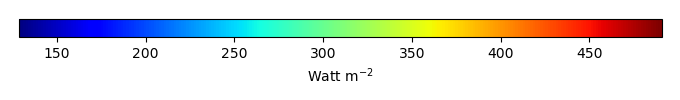MODEL MEANBIAS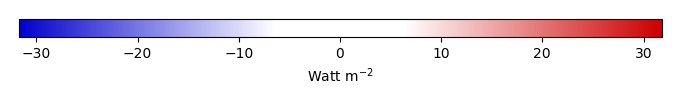BIAS SCORERMSE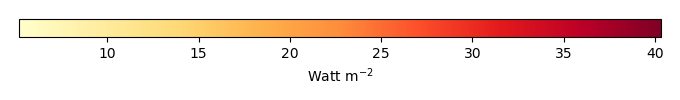RMSE SCOREBENCHMARK INTERANNUAL VARIABILITY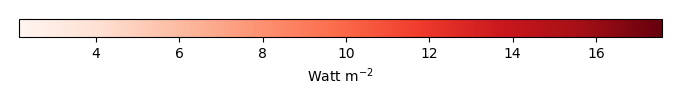MODEL INTERANNUAL VARIABILITYINTERANNUAL VARIABILITY SCOREBENCHMARK MAX MONTHMODEL MAX MONTHDIFFERENCE IN MAX MONTH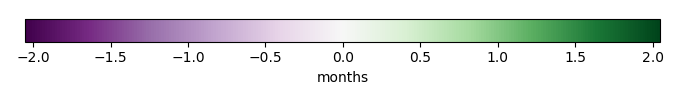SEASONAL CYCLE SCORESPATIAL TAYLOR DIAGRAMMODEL COLORS# Spatially integrated regional mean

MODEL COLORSREGIONAL MEANANNUAL CYCLEMONTHLY ANOMALYANNUAL CYCLE# All Models

BenchmarkCLM4CLM4.5CLM5# Data Information

creation_date: Mon Jun 30 23:35:20 PDT 2014

source_file: This product is generated from monthly 1 degree GEWEX SRB Radiation observations